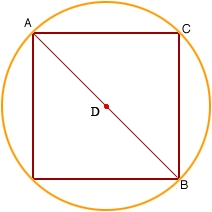# Calculate 39131

A circle describes a square with a side of 8 cm. Calculate the area of the rest of the circle if we cut out the square.

S =  36.531 cm2

### Step-by-step explanation:Did you find an error or inaccuracy? Feel free to write us. Thank you!

Tips for related online calculators
The Pythagorean theorem is the base for the right triangle calculator.

#### Grade of the word problem:

We encourage you to watch this tutorial video on this math problem: